## 14 Questions MCQ Test GATE Computer Science Engineering(CSE) 2023 Mock Test Series | Test: Deadlock- 2

Description
Attempt Test: Deadlock- 2 | 14 questions in 45 minutes | Mock test for Computer Science Engineering (CSE) preparation | Free important questions MCQ to study GATE Computer Science Engineering(CSE) 2023 Mock Test Series for Computer Science Engineering (CSE) Exam | Download free PDF with solutions
QUESTION: 1

### An operating system contains 3 user processes each requiring 2 units of resource R. The minimum number of units of R such that no deadlock will ever occur is

Solution:

Dead lock occurs when each of the 3 user processes hold one resource and make simultaneous demand for another. If there are 4 resources one of the 3 user processes will get the fourth instance of the resource and release one or both of the resource it is currently holding after using.

QUESTION: 2

### With a single resource, deadlock occurs

Solution:

Deadlock will never occur for a single resource.

QUESTION: 3

### A computer system has 6 tape drives, with 'n' processes competing for them. Each process may need 3 tape drives. The maximum value of ‘n’ for which the system is guaranteed to be deadlock free is

Solution:

2 process can never lead to deadlock as the peak time demand of 6 (3 + 3) tape drives can be satisfied. But 3 processes can lead to a deadlock if each hold 2 drives and then demand one more.

QUESTION: 4

Consider a system having 'm' resources of the same type. These resources are shared by 3 processes A, B, C, which have peak time demands of 3, 4, 6 respectively. The minimum value of ‘m’ that ensures that deadlock will never occur is

Solution:

Having 11 resources ensures that at least 1 process will have no pending request. This process after using will release the resources and so deadlock can never occur,
or m = (PA- 1 ) + (RB - 1 ) + (RC -1 ) + 1
= 2 + 3 + 5 + 1 = 11

QUESTION: 5

A system has 3 processes sharing 4 resources. If each process needs a maximum of 2 units then, deadlock

Solution:

At least one process will be holding 2 resources in case of a simultaneous demand from, all the processes. That process will release the 2 resources, thereby avoiding any possible deadlock.

QUESTION: 6

'm' processes share 'n' resources of the same type. The maximum need of each process does not exceed 'n' and the sum all their maximum needs is always less than m + n. In this set up deadlock

Solution:

Assume m = 5 and n = 10
That means, 5 processes are sharing 10 resources and worst case will be if everyone is demanding equal number of cases.
So, for deadlock to be there every process must be holding 2 resources and seeking 1 more resource. This will make total demand to 15, which is nothing but 10 + 5 (m + n).
However, as given maximum demand is always less than (m + n), so we can say there will never be a deadlock.

QUESTION: 7

Consider the resource allocation graph in figure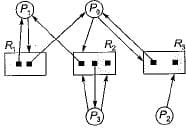​This system is in a deadlock state. This remark is

Solution:

The system is not in deadlock state, if the following sequence of fulfillment of request of the processes is made.
P2→ Po→ P1→ P3

QUESTION: 8

Consider:
P = { P1, P2,P3}
R = {R1, R2, R3, R4}
One instance of resource type R1 two instances of resource type R2, one instances of resource type R3 and three instances of resource type R4. The situation is P1 is holding one instance of R2 and waiting for an instance R1, P2 is holding an instance of R1 one instance of R2 and waiting for an instance of R3 and P3 is holding an instance of R3.

Solution: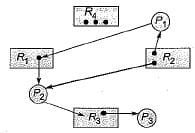Dead lock can not occure since first P3 will complete then P2 then P1 will complete.

QUESTION: 9

Match List-I with List-II select the correct answer using the codes given below the lists:
List-I
A. Inconsistency
D. Transit
List-II
1. Requires the recognition of unsafe states.
2. A message received by Pj from Pi that is not shown as sent by Pi
3. A message sent by Pi to Pj (mij) that does not belong to the set of messages received by Pj
4. Always requires the abortion of one of more executing processes.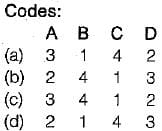Solution:

Inconsistency: message received by Pj from Pi that is not shown as sent by Pi. Deadlock resolution: always require the abortion of one or more processes. Deadlock avoidance: requires the recognition of unsafe state.
Transit: message sent by Pi to Pj that does not belong to the set of messages received by Pj.

QUESTION: 10

Consider the following system state: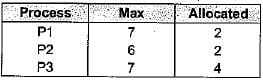Total resources are 11. The system will be in a safe state if

Solution: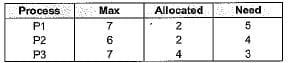If three additional resources are allocated P3 then its need becomes zero. And the system will get seven resources back from P3. These resources can be allocated to either P1 or P2 to become the system in safe state.

QUESTION: 11

The time complexity of Banker’s algorithm to avoid deadlock having n processes and m resources is

Solution:

The time complexity of the banker’s algorithm as a function of number n of processes and m of resources is O(n2xm).

QUESTION: 12

Consider a sytem with five processes P0 through P4 and three resource types A, B, C. Resource type A has 10 instances, resource type B has 5 instances, and resource type C has 7 instances. Suppose that, at time to, the following snapshot of the system has been taken: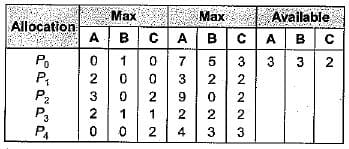Which of the following is the safe sequence for the above system?

Solution: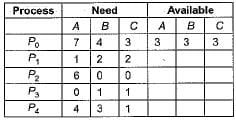<P1, P3, P4, P2, P0> is the safe sequence.

QUESTION: 13

Consider a system with three resources, tape drive, printer and disk drive. Each one of them are assigned a unique number:
R1 = F(Printer) = 12
R2 = F (Tape Drive) = 1
R3 = F(Disk Drive) = 4
Which of the following access patterns necessarily avoids deadlock?

Solution:

To avoid deadlock, we must request the devices in ascending order of enumeration. This ensures that circular wait does not hold. The correct sequence is 1, 4, 12, i.e. R2, R3, R1.

QUESTION: 14

​Which of the following is true?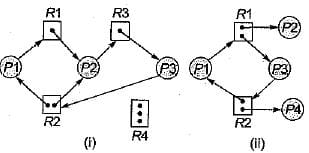Solution:

In (a) the processes P1, P2 and P3 are in deadlock state. P2 waiting on P3. Which is held by P3. P1 is waiting on ft, which is held by P2. While P3 in waiting on R2 which is held by P1 and P2
In (b) we can observe that P4 can release instance o f R2, which can be allocated to either P1 or P3, breaking the cycle. Deadlock does not occur in (b).Use Code STAYHOME200 and get INR 200 additional OFF Use Coupon Code